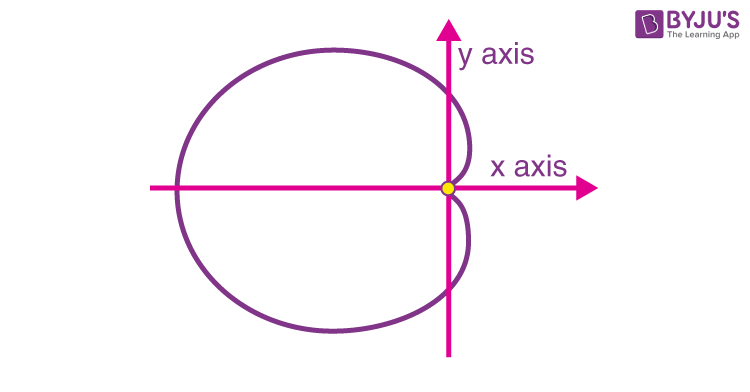Checkout JEE MAINS 2022 Question Paper Analysis : Checkout JEE MAINS 2022 Question Paper Analysis :

# Cardioid

A cardioid is a two-dimensional plane figure that has a heart-shaped curve. The word “cardioid” originated from a Greek word, which means “heart”. Hence, it is called a heart-shaped curve. The shape of a cardioid can be compared to the cross-section of an apple excluding its stalk. In Mathematics, we study different types of shapes and figures. There are many two-dimensional and three dimensional shapes studied in geometry. Cardioid is one of the important ones.

It is used frequently in higher Mathematics and also in various other fields. The shape is formed by tracing a point on the boundary of a circle, rolling onto another circle of the same radius. In this article, we will learn in detail about cardioid, its equation and several other concepts based on it.

## Definition of Cardioid

A cardioid is a heart-shaped plane figure. It is a curve which is defined as the locus of a point lying on the circumference of a circle that is rolling externally without any slip on the boundary of another circle of the same radius. See the figure here to understand how to draw a cardioid.In this way, a curve resembling the shape of a heart is formed. This curve is termed as cardioid. It is a form of a sinusoidal spiral. This curve is the inverse of a parabola having focus at the centre of inversion. A cardioid does have exactly 3 parallel tangents with any particular gradient. It has a cusp (formed by the intersection of two branches of a curve). The length of the passing through the cusp of the cardioid is 4a, where “a” be the circle radius.

## Equation of the Cardioid

The equation of the cardioid is represented into polar form, which can also be converted later into the cartesian coordinate system. Let us check both the forms here.

### Polar Equation of Cardioid

The polar equation of the horizontal cardioid is given by;

 r = a(1 ± cosθ)

Similarly, the polar equation of the vertical cardioid is given by:

 r = a(1 ± sinθ)

Where a is the radius of the tracing circle and θ is the polar angle.

### Cartesian Equation of Cardioid

The cartesian form of the cardioid equation is given by;

 (x2+y2+ax)2=a2(x2+y2)

Whose parametric equations are as follows:

x = a cos t (1 – cos t)

y = a sin t (1 – cos t)

## Graph of Cardioid

A cardioid is a shape, defined in two dimensions, that looks like the shape of a heart. The cardioid is formed by following the path of a point on a rolling circle over another fixed circle of the same radius. There is one cusp in the cardioid. Usually, a Cardioid is represented in the polar coordinate system, but it may also be shown in the Cartesian system. The graph of a cardioid is demonstrated below:## Area of Cardioid

The area of the cardioid is the region enclosed by it in a two-dimensional plane. The formula to calculate its area depends on the radius of the tracing circle.

 Area = 6 π a2

Where

“a” is the radius of the tracing circle.

So from the formula, we can see, the area of a cardioid is six times equal to the area of the tracing circle.

### Length of the Arc

The total length of the arc formed by a cardioid is calculated by;

 Arc Length = 16a

## Examples of Cardioid

Example 1:

A cardioid is given by the equation r = 2 (1 + cos θ). Find its area.

Solution:

Here, a = 2.

The area of the cardioid is given by:

Area = 6 π a2
Area = 6 π 4

Area = 24 π sq unit.

Example 2:

Calculate the area and arc length of the cardioid, which is given by the following equation r = 7 (1 + cos θ).

Solution:

Here, a = 7

The formula for area of cardioid is given by :

A = 6 x 22/7 x 72

A = 6 x 22 x 7

A = 924 sq unit.

The arc length of the cardioid is calculated by :

L = 16 a = 16 x 7 = 112 unit.

Example 3:

If a circle with equation r = 3 sin θ and a cardioid whose equation is r = 1 + sin θ intersect each other. Find the points where these curves may intersect.

Solution:

To find the intersection points of the given curve, we must solve them.

Given, r = 3 sin θ r = 1 + sin θ

Therefore, 3 sin θ = 1 + sin θ

2 sin θ = 1

sin θ = 1/2

θ = π/6 and 5π/6

These intersections lie in the first and second quadrants.

Stay tuned with BYJU’S – The Learning App and download the app to learn all Maths-related concepts easily by exploring more videos.

## Frequently Asked Questions on Cardioid

### What is cardioid in Maths?

A cardioid is a heart-shaped plane figure that is defined as the locus of a point lying on the circumference of a circle that is rolling externally without any slip on the boundary of another circle of the same radius.

### What is the polar equation of the horizontal cardioid?

The polar equation of the horizontal cardioid is r = a(1 ± cosθ).

### What is the polar equation of the vertical cardioid?

The polar equation of the vertical cardioid is r = a(1 ± sinθ)

### What is the area inside the cardioid “C”?

The area inside the cardioid “C” is 6πa2, Where “a” is the radius of the tracing circle.

### What is the formula to calculate the total length of the arc formed by a cardioid?

The formula to calculate the total arc length formed by a cardioid is 16a provided that “a” is the radius of the tracing circle.